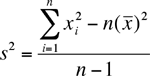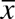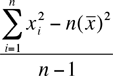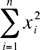Quandaries and Queries hi I must keep statistical data (mean and variance) in 3 granularity levels depending on the age of the data (daily for older than 1 year, hourly for older than 1 month and quarter-hour for older than 1 day). How can I calculate the resulting variance from a set of variances previously calculated supposing I have the count and mean for each member of the set? thank you Hi Carlos, There a number of equivalent expressions for the variance. The one I want to use iswhereis the mean of the x-values. I want to change the notation to make it easier to type. Let V(n) =M(n) =S(n) =The expression for the variance is then V(n) = (S(n) - n(M(n))2)/(n + 1) Assume that you have the calculations for n x-values so you know n, V(n), M(n), and you receive a new x-value, xn+1. M(n) is the sum of the previous x-values divided by n so you can recover the sum of the previous x-values by nM(n). Thus M(n+1) = (nM(n) + xn+1)/(n + 1) To evaluate V(n+1) all that remains is to find S(n+1). You know V(n), M(n) and n so solve V(n) = (S(n) - n(M(n))2)/(n + 1) for S(n). Now calculate S(n+1) = S(n) + xn+12 and then V(n+1). Andrei and Penny Go to Math Central